# MA.7.NSO.1.2Export Print
Rewrite rational numbers in different but equivalent forms including fractions, mixed numbers, repeating decimals and percentages to solve mathematical and real-world problems.

### Examples

Justin is solving a problem where he computes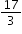and his calculator gives him the answer 5.6666666667. Justin makes the statement that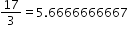; is he correct?
General Information
Subject Area: Mathematics (B.E.S.T.)
Strand: Number Sense and Operations
Status: State Board Approved

## Benchmark Instructional Guide

### Terms from the K-12 Glossary

• Rational Number

### Vertical Alignment

Previous Benchmarks

Next Benchmarks

### Purpose and Instructional Strategies

In grade 6, students rewrote positive rational numbers in different but equivalent forms as long as the decimal form is terminating. This expectation expands to all rational numbers in grade 7, including those with repeating decimals, as well as using this skill to solve mathematical and real-world problems. In grade 8, students will learn about irrational numbers as well as working to plot, order and compare rational and irrational numbers.
• When solving problems with numbers written in various forms, students must be able to convert between these forms to perform operations or make comparisons (MTR.2.1).
• Students should begin to develop charts, like the one below, that allow them to find patterns within the different forms of rational numbers. Students should have common fractions, decimals and percentages at their disposal in order to move to ones that are more difficult to determine.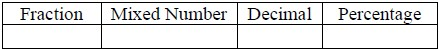• Students should have practice with and without the use of technology to rewrite rational numbers in different but equivalent forms.
• Students should work with simple problems to showcase how truncating repeated decimals may result in incorrect solutions.
• For example, using the fractional value of $\frac{\text{1}}{\text{3}}$ may provide a more precise answer than using the truncated decimal of 0.33.
• Students should use reasonableness to determine if it is appropriate to use a specific equivalent form over another one when problem solving (MTR.6.1).

### Common Misconceptions or Errors

• Students may not differentiate between terminating decimals, repeating decimals and rounded decimals, and they may not use them appropriately within the given contexts.
• Students may incorrectly truncate repeating decimals when problem solving.
• Students may incorrectly divide when the quotient is not a whole number.
• For example, students may use the remainder of a problem as a decimal representation.

### Strategies to Support Tiered Instruction

• Instruction includes the use of estimation to find the approximate decimal value of a fraction or mixed number before rewriting in decimal form to help with correct placement of the decimal point.
• Teacher provides opportunities for students to explore and discuss the differences between repeating and truncated decimals and the impact of truncating repeating decimals when solving problems.
• For example, provide students with the equation $y$= $\frac{\text{1}}{\text{3}}$$x$ and have them create a table of values comparing using $\frac{\text{1}}{\text{3}}$, 0.3, 0.333 and 0.33333 as the constant of proportionality. Students can discuss the differences in $y$-values and importance of using exact values in some cases and approximate values in others.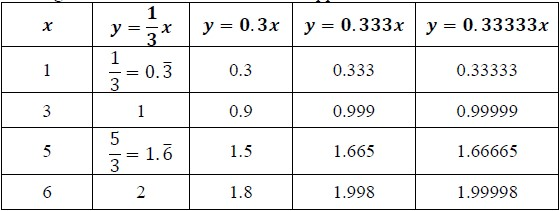• Instruction includes co-creating a graphic organizer to highlight the differences between terminating decimals, repeating decimals, and rounded decimals.

Convert each of the following to an equivalent form in order to compare their values.
$\frac{\text{1}}{\text{5}}$ − 0.4  65%  − 2$\frac{\text{1}}{\text{3}}$ 5.75  $\frac{\text{9}}{\text{7}}$ 123%  2.3¯
• Part A. Graph the numbers on a number line to determine increasing order.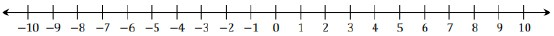• Part B. Robin plotted her number line using all decimals, whereas Courtney plotted them using the original forms. Describe why both would be acceptable answers.

Instructional Task 2 (MTR.2.1, MTR.4.1, MTR.5.1)

Complete the table to identify equivalent forms of each number. Explain how you approached your solutions. Prompting questions: What patterns did you use? How did you start? Which values in the table are you most comfortable in starting with?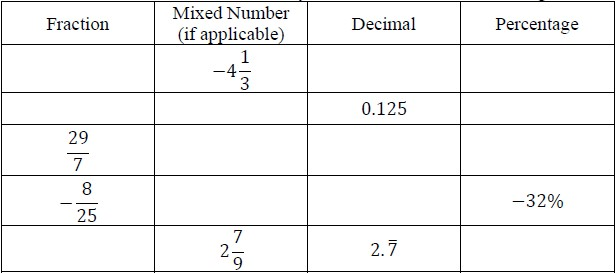### Instructional Items

Instructional Item 1
All of the students in first period were given a glue stick to help build their interactive notebook. Benny said he has already used $\frac{\text{2}}{\text{3}}$ of his glue while Juniper has used 70% of hers. Which student has the most glue remaining for their notebooks?

Instructional Item 2
Ishana manages a corner store and wishes to give a discount to her customers for the holiday. If she subtracts 0.15 of the cost of any item in the store, what percent should her sale sign promote?

Instructional Item 3
Write three equivalent forms for 5$\frac{\text{7}}{\text{8}}$.

*The strategies, tasks and items included in the B1G-M are examples and should not be considered comprehensive.

## Related Courses

This benchmark is part of these courses.
1200400: Foundational Skills in Mathematics 9-12 (Specifically in versions: 2014 - 2015, 2015 - 2022, 2022 and beyond (current))
1205040: M/J Grade 7 Mathematics (Specifically in versions: 2014 - 2015, 2015 - 2022, 2022 and beyond (current))
1205050: M/J Accelerated Mathematics Grade 7 (Specifically in versions: 2014 - 2015, 2015 - 2020, 2020 - 2022, 2022 and beyond (current))
1204000: M/J Foundational Skills in Mathematics 6-8 (Specifically in versions: 2014 - 2015, 2015 - 2022, 2022 and beyond (current))
7812020: Access M/J Grade 7 Mathematics (Specifically in versions: 2014 - 2015, 2015 - 2018, 2018 - 2019, 2019 - 2022, 2022 and beyond (current))

## Related Access Points

Alternate version of this benchmark for students with significant cognitive disabilities.
MA.7.NSO.1.AP.2: Rewrite positive rational numbers in different but equivalent forms such as fractions, mixed numbers, repeating decimals and/or percentages to solve problems.

## Related Resources

Vetted resources educators can use to teach the concepts and skills in this benchmark.

## Educational Game

Fraction Quiz:

Test your fraction skills by answering questions on this site. This quiz asks you to simplify fractions, convert fractions to decimals and percentages, and answer algebra questions involving fractions. You can even choose difficulty level, question types, and time limit.

Type: Educational Game

## Formative Assessments

Find Decimal Using Long Division:

Students are asked to use long division to convert four different fractions to equivalent decimals and to identify those that are rational.

Type: Formative Assessment

Quotients of Integers:

Students are given an integer division problem and asked to identify fractions which are equivalent to the division problem.

Type: Formative Assessment

Fraction to Decimal Conversion:

Students are given a fraction to convert to a decimal and are asked to determine if the decimal repeats.

Type: Formative Assessment

## Lesson Plans

Independent Compound Probability:

During this lesson, students will use Punnett Squares to determine the probability of an offspring's characteristics.

Type: Lesson Plan

Fast Food Frenzy:

In this activity, students will engage critically with nutritional information and macronutrient content of several fast food meals. This is an MEA that requires students to build on prior knowledge of nutrition and working with percentages.

Type: Lesson Plan

Predicting the decimal equivalent for a fraction - terminating or repeating?:

This lesson encourages students to make an important discovery. Will a given fraction yield a terminating or repeating decimal? Discussion includes why knowing this is important. The lesson is structured to allow exploration, discovery, and summarization.

Type: Lesson Plan

## STEM Lessons - Model Eliciting Activity

Fast Food Frenzy:

In this activity, students will engage critically with nutritional information and macronutrient content of several fast food meals. This is an MEA that requires students to build on prior knowledge of nutrition and working with percentages.

## MFAS Formative Assessments

Find Decimal Using Long Division:

Students are asked to use long division to convert four different fractions to equivalent decimals and to identify those that are rational.

Fraction to Decimal Conversion:

Students are given a fraction to convert to a decimal and are asked to determine if the decimal repeats.

Quotients of Integers:

Students are given an integer division problem and asked to identify fractions which are equivalent to the division problem.

## Student Resources

Vetted resources students can use to learn the concepts and skills in this benchmark.

## Educational Game

Fraction Quiz:

Test your fraction skills by answering questions on this site. This quiz asks you to simplify fractions, convert fractions to decimals and percentages, and answer algebra questions involving fractions. You can even choose difficulty level, question types, and time limit.

Type: Educational Game

## Parent Resources

Vetted resources caregivers can use to help students learn the concepts and skills in this benchmark.